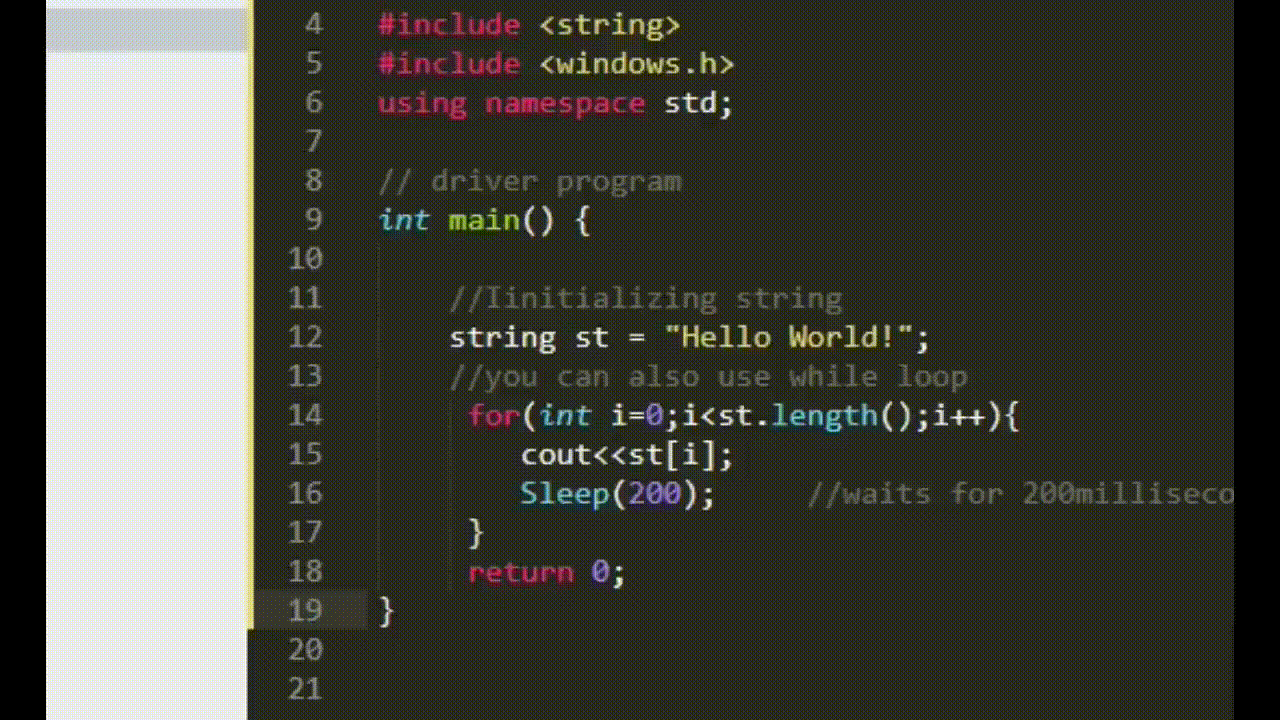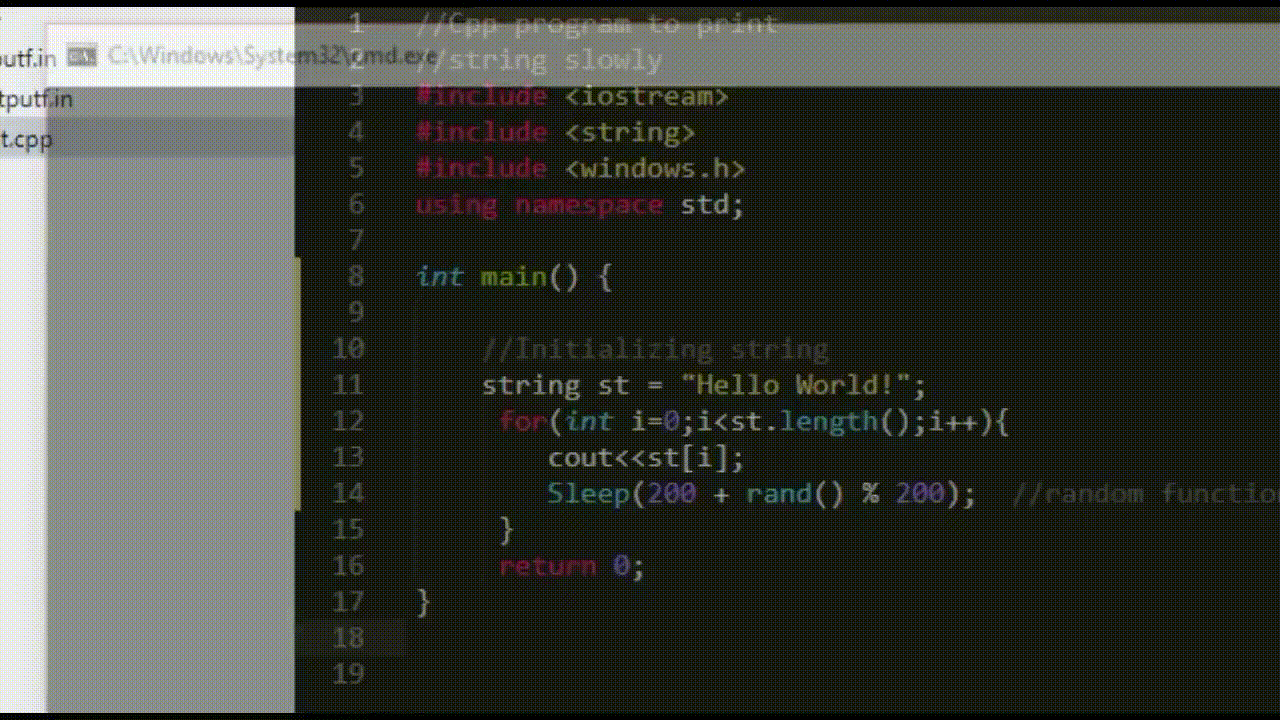# Program to display characters slowly on the console in C++

• Difficulty Level : Basic
• Last Updated : 28 Apr, 2021

The task is to write a C++ program that displays the characters of the given string slowly on the console.

Approach: The given problem can be solved by using the sleep() function in C++.

• <windows.h> for windows
• <unistd.h> for Linux

Syntax:

Sleep(time_in_milliseconds)

Random Function: The rand() function in C++ generates random numbers in the range [0, RAND_MAX]. If the random numbers are generated using the rand() function without first calling srand() the program will create the same sequence of numbers each time it is executed.

Syntax:

rand(void)

Program 1:

Below is the implementation to display characters slowly on the console in C++ using the sleep function:

## C++

 `// C++ program for the above approach`` ` `#include ``#include ``#include ``using` `namespace` `std;`` ` `// Driver Code``int` `main()``{``    ``// Initialize the string``    ``string S = ``"Hello World!"``;`` ` `    ``// Traverse the given string S``    ``for` `(``int` `i = 0; i < S[i]; i++) {`` ` `        ``cout << S[i];`` ` `        ``// Waits for 200 milliseconds``        ``Sleep(200);``    ``}`` ` `    ``return` `0;``}`

Output:Program 2:

Below is the implementation to display characters slowly on the console in C++ using the sleep function and the random function:

## C++

 `// C++ program for the above approach`` ` `#include ``#include ``#include ``using` `namespace` `std;`` ` `// Driver Code``int` `main()``{``    ``string S = ``"Hello World!"``;`` ` `    ``for` `(``int` `i = 0; i < S.length(); i++) {``        ``cout << S[i];`` ` `        ``// random function generates``        ``// random values``        ``Sleep(200 + ``rand``() % 200);``    ``}`` ` `    ``return` `0;``}`

Output:My Personal Notes arrow_drop_up